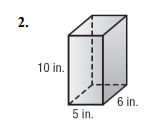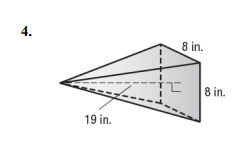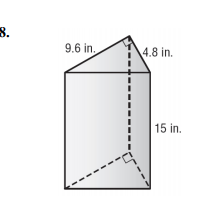Volume of Pyramids and Prisms
starstarstarstarstarstarstarstarstarstar
by Judy Riggle
| 60 Questions1
1 pt
What is this shape?
Rectangular Prism
Rectangular Pyramid
Triangular Prism
Triangular Pyramid
2
1 pt
What formula would you use to find the volume for this shape?
V=Bh
V=1/3Bh
3
1 pt
What is the Base of this shape?
square
rectangle
triangle
4
1 pt
What formula would you use the find B?
5
1 pt
6
1 pt
What is h for this shape?
7
1 pt
What is B for this shape?
8
1 pt
What is B for this shape?
9
1 pt
What is the Volume of the shape?10
1 pt
What is this shape?
Rectangular Prism
Rectangular Pyramid
Triangular Prism
Triangular Pyramid
11
1 pt
What formula would you use to find the volume for this shape?
V=Bh
V=1/3Bh
12
1 pt
What is the Base of this shape?
Rectangle
Square
Triangle
13
1 pt
14
1 pt
15
1 pt
What formula would you use the find B?
A=1/2bh
A=lxw
16
1 pt
17
1 pt
What is B for this shape?
18
1 pt
What is the volume of the shape?19
1 pt
What is this shape?
Rectangular Prism
Rectangular Pyramid
Triangular Prism
Triangular Pyramid
20
1 pt
What formula would you use to find the volume for this shape?
V=1/3Bh
V=Bh
21
1 pt
What is the Base of this shape?
Triangle
Rectangle
Square
Pentagon
22
1 pt
What formula would you use the find B?
A=1/2bh
A=lxw
23
1 pt
What is B for this shape?
24
1 pt
What is the Volume of the shape?25
1 pt
What is this shape?
Rectangular Prism
Rectangular Pyramid
Triangular Prism
Triangular Pyramid
26
1 pt
What formula would you use to find the volume for this shape?
V=1/3Bh
V=Bh
27
1 pt
What is the Base of this shape?
Triangle
Square
Rectangle
28
1 pt
What formula would you use the find B?
A=1/2bh
A=lxw
29
1 pt
What is B for this shape?
30
1 pt
What is the Volume of the shape?31
1 pt
What is this shape?
Rectangular Prism
Rectangular Pyramid
Triangular Prism
Triangular Pyramid
32
1 pt
What formula would you use to find the volume for this shape?
V=1/3Bh
V=Bh
33
1 pt
What is the Base of this shape?
Triangle
Rectangle
Square
34
1 pt
What formula would you use the find B?
A=1/2bh
A=lxw
35
1 pt
What is B for this shape?
36
1 pt
What is the Volume of the shape?37
1 pt
What is this shape?
Rectangular Prism
Rectangular Pyramid
Triangular Prism
Triangular Pyramid
38
1 pt
What formula would you use to find the volume for this shape?
V=1/3Bh
V=Bh
39
1 pt
What is the Base of this shape?
Rectangle
Square
Triangle
40
1 pt
What formula would you use the find B?
A=1/2bh
A=lxw
41
1 pt
What is B for this shape?
42
1 pt
What is the Volume of the shape?43
1 pt
What is this shape?
Rectangular Prism
Rectangular Pyramid
Triangular Prism
Triangular Pyramid
44
1 pt
What formula would you use to find the volume for this shape?
V=1/3Bh
V=Bh
45
1 pt
What is the Base of this shape?
Triangle
Square
Rectangle
46
1 pt
What formula would you use the find B?
A=Lxw
A=1/2bh
47
1 pt
What is B for this shape?
48
1 pt
What is the Volume of the shape?49
1 pt
What is this shape?
Rectangular Prism
Rectangular Pyramid
Triangular Prism
Triangular Pyramid
50
1 pt
What formula would you use to find the volume for this shape?
V=1/3Bh
V=Bh
51
1 pt
What is the Base of this shape?
Triangle
Square
Rectangle
52
1 pt
What formula would you use the find B?
A=1/2bh
A=lxw
53
1 pt
What is B for this shape?
54
1 pt
What is the Volume of the shape?55
1 pt
What is this shape?
Rectangular Prism
Rectangular Pyramid
Triangular Prism
Triangular Pyramid
56
1 pt
What formula would you use to find the volume for this shape?
V=1/3Bh
V=Bh
57
1 pt
What is the Base of this shape?
Triangle
Rectangle
Square
58
1 pt
What formula would you use the find B?
A=1/2bh
A=lxw
59
1 pt
What is B for this shape?
60
1 pt
What is the Volume of the shape?